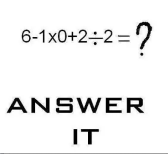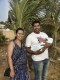There is a Facebook post going around and I thought it would be a fun Friday Challenge edition.Then put in the formula into Excel and see what you get.

Let me know what you think it should be and what answer you got from Excel in the comments below.

Hopefully, they match 🙂

Steve=True

PS: Sorry if this causes you problems at home.  It seems no too answers are the same 🙂 when two people look at this question.

PSPS: My answer matched Excel 🙂 so I must be right 🙂 LOL

1.Pete

2.Ken G

7 for me and Excel.
Order of operations.
Multiply and divide first from left to right.
Then add and subtract from left to right.
When I did real math, I really loved my HP – RPN (reverse polish notation) calculator.

•SteveEqualsTrue

Hi Ken, I could never get my head wrapped around it. I have seen some posts that state because there are no ( ) then you go left to right. Then you would get a different answer like “1”. I saw other posts of “5”. So I posted this because I think Excel gets it right. 🙂 And it matched my answer, so that makes me right, right?

• # If you found the website and tutorials helpful, please consider donating to keep the lights on.

## Donate with PayPal here:•Leonid

As I understand 1×0 in your formula means 1*0. Otherwise result will be a “#NAME?” error. So, the answer depends on how to interpret the last operator and it’s not as simple as it seems. If it is 2/2 then the formula result is 7. But if it’s 2:2 (which means row 2:2 because “:” works here as a reference operator) then it is 6 with a simple Enter. With using Shift+Ctrl+Enter result depends on a value in A2. If it’s numeric the result will be 6 plus that value(just 6 if it’s empty), otherwise we’ll get “#value!” error. If we try to enter such formula in the row 2 itself, but not in A2, we’ll receive circular reference warning and result 0. And if we try to Shift+Ctrl+Enter it in the cell A2 Excel can’t calculate the formula.

•Ju1ieM

=((6-(1*0))+2)/2=4

•SteveEqualsTrue

Interesting answer JulieM. I have also seen this answer from people on Facebook: 6-1×0+2/2 = 5×0+2/2 = 0+2/2 = 1. Mine answer was: 6-(1*0)+(2/2) = 6-0+1 = 7 Excel told me the answer was 7 as well, so I must be right, right 🙂 Thanks Steve=True

•Leonid

Then why not
=(6-(1*0+2))/2=2
or
=(6-1)*0+2/2=1
🙂

• # If you found the website and tutorials helpful, please consider donating to keep the lights on.

## Donate with PayPal here:•Piety Fernandes

BODMAS rule studied in school for solving such problems.Bracket,Open,Division,Multiplication, Addition and then Subtraction. Follow this sequence and the answer is 7 which matches with Excel.

•SteveEqualsTrue

Hi Piety,

You are correct. That is the way it should be calculated, but this problem seems to throw people for a loop and they add in their own ( ) that don’t exist. Excel to the rescue 🙂 Thanks again!

Steve=True

•Niraj Bahadur Pal

=(6-1)*0+(2/2), and My answer is coming 1. Excel is giving me the right answer. Right.

Thank you,
Niraj

•SteveEqualsTrue

Yes Excel is giving you the right answer. It is an order of operations question that Excel can help determine the answer. Thanks Steve=True

•Niraj Bahadur Pal

it can be calculated too like this 6-(1*0)+(2/2) = 6-0+1 = 7. But again, depends how you look at the solution if this is not defined.

Thanks,
Niraj

•SteveEqualsTrue

Hi Niraj, if you enter the formula exactly as posted, Excel will give you the right answer. You should not add any extra ( ) that are not already there as that changes the order of operation and would give you a different answer. Look at the many ways that Leonid showed in the comments [if you add extra ()] around numbers. Thanks Steve=True